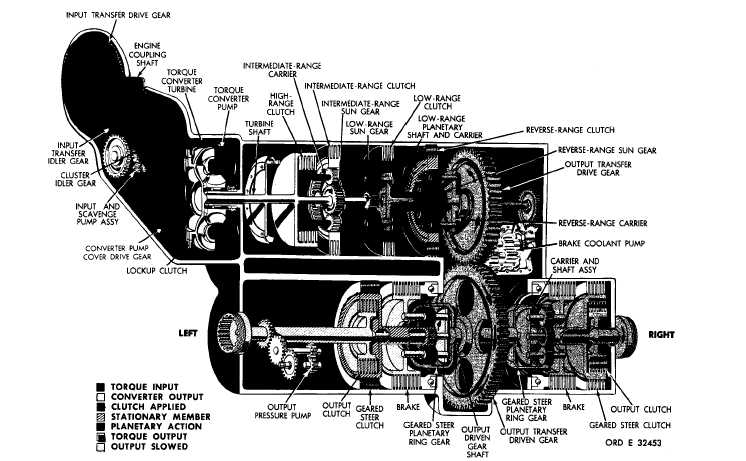Custom SearchP A R   5  8 T O R Q U E     P A T HS C H A P   2,   SEC  V 58. Figure    34.    Reverse    2-gear,    full-left-steer    torque    path,converter    operation TORQUE   PATH - REVERSE   2   GEAR, train   outputs,   the   torque   path   is   the   same   as FULL - LEFT    STEER,    CONVERTER d e s c r i b e d    f o r    t h i r d    g e a r    ( p a r .    5 b ,      and   d , OPERATION  (fig.  34) above)   except   that   rotations   are   opposite   and that   the   steering   action   is   on   the   left   side   of The   torque   path   from   the   engine   to   the the   power   train   instead   of   the   right   side   as   de- steer   planetary   ring   gears   is   identical   to   that scribed   for   third   gear. Refer   to   T A B U L A T ED for   reverse   1   gear   as   described   in   par.   51a, D A T A ,   p a r .    7 ,    f o r    g e a r    r a t i o s    a p p l i c a b l e    to  and  ,   above.   From   this   point,   to   the   power r e v e r s e    2    g e a r ,    l e f t    s t e e r. 61Integrated Publishing, Inc. - A (SDVOSB) Service Disabled Veteran Owned Small Business